Next: Worked example 8.1: Balancing Up: Rotational motion Previous: The physics of baseball

## Combined translational and rotational motion

In Sect. 4.7, we analyzed the motion of a block sliding down a frictionless incline. We found that the block accelerates down the slope with uniform acceleration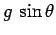, whereis the angle subtended by the incline with the horizontal. In this case, all of the potential energy lost by the block, as it slides down the slope, is converted into translational kinetic energy (see Sect. 5). In particular, no energy is dissipated.

There is, of course, no way in which a block can slide over a frictional surface without dissipating energy. However, we know from experience that a round object can roll over such a surface with hardly any dissipation. For instance, it is far easier to drag a heavy suitcase across the concourse of an airport if the suitcase has wheels on the bottom. Let us investigate the physics of round objects rolling over rough surfaces, and, in particular, rolling down rough inclines.

Consider a uniform cylinder of radius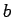rolling over a horizontal, frictional surface. See Fig. 83. Letbe the translational velocity of the cylinder's centre of mass, and letbe the angular velocity of the cylinder about an axis running along its length, and passing through its centre of mass. Consider the point of contact between the cylinder and the surface. The velocity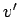of this point is made up of two components: the translational velocity, which is common to all elements of the cylinder, and the tangential velocity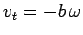, due to the cylinder's rotational motion. Thus,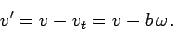(396)

Suppose that the cylinder rolls without slipping. In other words, suppose that there is no frictional energy dissipation as the cylinder moves over the surface. This is only possible if there is zero net motion between the surface and the bottom of the cylinder, which implies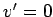, or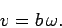(397)

It follows that when a cylinder, or any other round object, rolls across a rough surface without slipping--i.e., without dissipating energy--then the cylinder's translational and rotational velocities are not independent, but satisfy a particular relationship (see the above equation). Of course, if the cylinder slips as it rolls across the surface then this relationship no longer holds.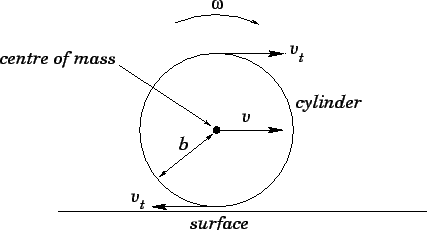Consider, now, what happens when the cylinder shown in Fig. 83 rolls, without slipping, down a rough slope whose angle of inclination, with respect to the horizontal, is. If the cylinder starts from rest, and rolls down the slope a vertical distance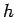, then its gravitational potential energy decreases by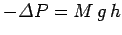, where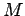is the mass of the cylinder. This decrease in potential energy must be offset by a corresponding increase in kinetic energy. (Recall that when a cylinder rolls without slipping there is no frictional energy loss.) However, a rolling cylinder can possesses two different types of kinetic energy. Firstly, translational kinetic energy: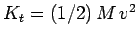, whereis the cylinder's translational velocity; and, secondly, rotational kinetic energy:, whereis the cylinder's angular velocity, and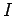is its moment of inertia. Hence, energy conservation yields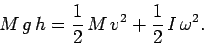(398)

Now, when the cylinder rolls without slipping, its translational and rotational velocities are related via Eq. (397). It follows from Eq. (398) that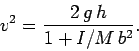(399)

Making use of the fact that the moment of inertia of a uniform cylinder about its axis of symmetry is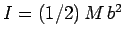, we can write the above equation more explicitly as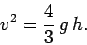(400)

Now, if the same cylinder were to slide down a frictionless slope, such that it fell from rest through a vertical distance, then its final translational velocity would satisfy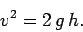(401)

A comparison of Eqs. (400) and (401) reveals that when a uniform cylinder rolls down an incline without slipping, its final translational velocity is less than that obtained when the cylinder slides down the same incline without friction. The reason for this is that, in the former case, some of the potential energy released as the cylinder falls is converted into rotational kinetic energy, whereas, in the latter case, all of the released potential energy is converted into translational kinetic energy. Note that, in both cases, the cylinder's total kinetic energy at the bottom of the incline is equal to the released potential energy.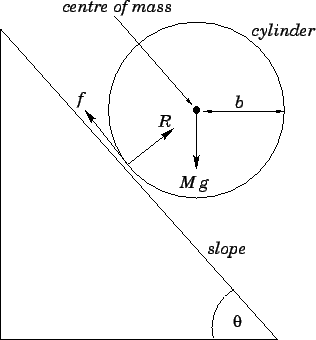Let us examine the equations of motion of a cylinder, of massand radius, rolling down a rough slope without slipping. As shown in Fig. 84, there are three forces acting on the cylinder. Firstly, we have the cylinder's weight,, which acts vertically downwards. Secondly, we have the reaction,, of the slope, which acts normally outwards from the surface of the slope. Finally, we have the frictional force,, which acts up the slope, parallel to its surface.

As we have already discussed, we can most easily describe the translational motion of an extended body by following the motion of its centre of mass. This motion is equivalent to that of a point particle, whose mass equals that of the body, which is subject to the same external forces as those that act on the body. Thus, applying the three forces,,, and, to the cylinder's centre of mass, and resolving in the direction normal to the surface of the slope, we obtain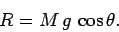(402)

Furthermore, Newton's second law, applied to the motion of the centre of mass parallel to the slope, yields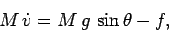(403)

where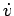is the cylinder's translational acceleration down the slope.

Let us, now, examine the cylinder's rotational equation of motion. First, we must evaluate the torques associated with the three forces acting on the cylinder. Recall, that the torque associated with a given force is the product of the magnitude of that force and the length of the level arm--i.e., the perpendicular distance between the line of action of the force and the axis of rotation. Now, by definition, the weight of an extended object acts at its centre of mass. However, in this case, the axis of rotation passes through the centre of mass. Hence, the length of the lever arm associated with the weightis zero. It follows that the associated torque is also zero. It is clear, from Fig. 84, that the line of action of the reaction force,, passes through the centre of mass of the cylinder, which coincides with the axis of rotation. Thus, the length of the lever arm associated withis zero, and so is the associated torque. Finally, according to Fig. 84, the perpendicular distance between the line of action of the friction force,, and the axis of rotation is just the radius of the cylinder,--so the associated torque is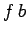. We conclude that the net torque acting on the cylinder is simply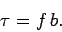(404)

It follows that the rotational equation of motion of the cylinder takes the form,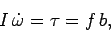(405)

whereis its moment of inertia, and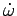is its rotational acceleration.

Now, if the cylinder rolls, without slipping, such that the constraint (397) is satisfied at all times, then the time derivative of this constraint implies the following relationship between the cylinder's translational and rotational accelerations: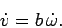(406)

It follows from Eqs. (403) and (405) that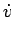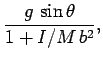(407)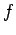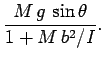(408)

Since the moment of inertia of the cylinder is actually, the above expressions simplify to give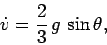(409)

and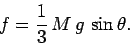(410)

Note that the acceleration of a uniform cylinder as it rolls down a slope, without slipping, is only two-thirds of the value obtained when the cylinder slides down the same slope without friction. It is clear from Eq. (403) that, in the former case, the acceleration of the cylinder down the slope is retarded by friction. Note, however, that the frictional force merely acts to convert translational kinetic energy into rotational kinetic energy, and does not dissipate energy.

Now, in order for the slope to exert the frictional force specified in Eq. (410), without any slippage between the slope and cylinder, this force must be less than the maximum allowable static frictional force,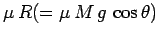, where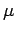is the coefficient of static friction. In other words, the condition for the cylinder to roll down the slope without slipping is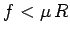, or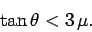(411)

This condition is easily satisfied for gentle slopes, but may well be violated for extremely steep slopes (depending on the size of). Of course, the above condition is always violated for frictionless slopes, for which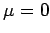.

Suppose, finally, that we place two cylinders, side by side and at rest, at the top of a frictional slope of inclination. Let the two cylinders possess the same mass,, and the same radius,. However, suppose that the first cylinder is uniform, whereas the second is a hollow shell. Which cylinder reaches the bottom of the slope first, assuming that they are both released simultaneously, and both roll without slipping? The acceleration of each cylinder down the slope is given by Eq. (407). For the case of the solid cylinder, the moment of inertia is, and so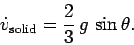(412)

For the case of the hollow cylinder, the moment of inertia is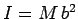(i.e., the same as that of a ring with a similar mass, radius, and axis of rotation), and so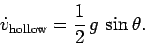(413)

It is clear that the solid cylinder reaches the bottom of the slope before the hollow one (since it possesses the greater acceleration). Note that the accelerations of the two cylinders are independent of their sizes or masses. This suggests that a solid cylinder will always roll down a frictional incline faster than a hollow one, irrespective of their relative dimensions (assuming that they both roll without slipping). In fact, Eq. (407) suggests that whenever two different objects roll (without slipping) down the same slope, then the most compact object--i.e., the object with the smallest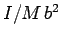ratio--always wins the race.Next: Worked example 8.1: Balancing Up: Rotational motion Previous: The physics of baseball
Richard Fitzpatrick 2006-02-02